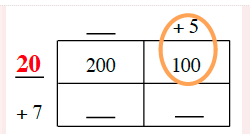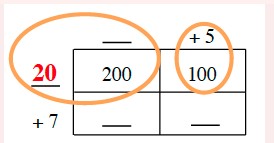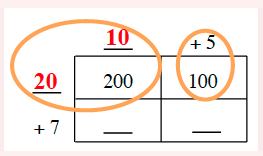### Home > CC2MN > Chapter 3 > Lesson 3.1.1 > Problem3-11

3-11.

Copy and complete the generic rectangle below. What multiplication problem does it represent and what is the product?

 _____ $+5$ _____ $200$ $100$ $+7$ _____ _____

2 by 2 generic rectangle edges labeled as follows: Top edge left, blank, top edge right, + 5. Left edge top, blank, left edge bottom, + 7. Interior area labeled as follows: top left, 200, top right, 200, bottom left, blank, bottom right, blank.

You know that $5(\text{something}) = 100$. What is that something?

Added to the rectangle: left edge top is 20. A circle around +5 and 100.

You know that $20(\text{something}) = 200$. What is that something?

Circle added around left edge top, 20, top edge left ,blank, and top left interior, 200.

Complete the rest of the square using multiplication. 2 by 2 generic rectangle: total length sections, 10 in red and positive 5, total width sections, 20 in red and positive 7. Inside rectangle areas: top row 200 and 100. Bottom row both blank.